Three Unusual Dice    |  Double Whammy    |   Efron Dice    |   Three Player Games  |   Grime Dice  |   A Gambling Game

# Non-transitive Dice

## Three Unusual Dice

Here is a game you can play with a friend. It is a game for two players, with a set of three dice. These dice are not typical dice however, because instead of having the values 1 to 6, they display various unusual values.

Each player picks a die. The two dice are then rolled together and whoever gets the highest value wins. Seems fair enough yet, in a game of say ten rolls, you will always be able to pick a die with a better chance of winning - no matter which die your friend chooses. And you can make these dice at home right now.

Here is the set of three special dice: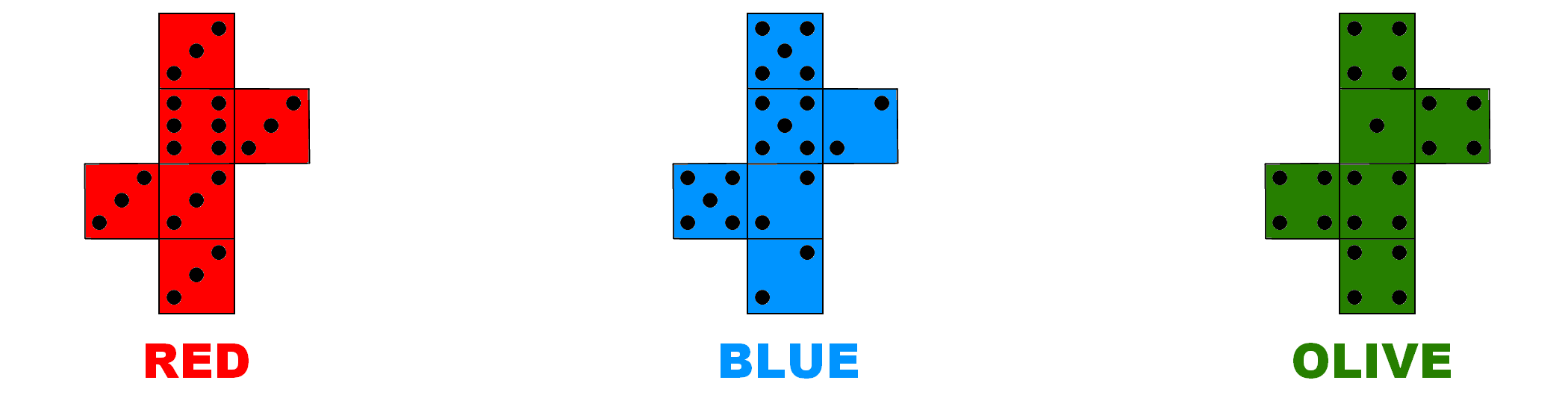Each die has six faces but only two values, as follows;

Red: 3 3 3 3 3 6
Blue: 2 2 2 5 5 5
Olive: 1 4 4 4 4 4

It can be shown (see below) that in the long run the Red die beats the Blue die, and that the Blue die beats the Olive die. So it appears the Red die is the strong die and the Olive die is the weak die. So you might expect the Red die to beat the Olive die;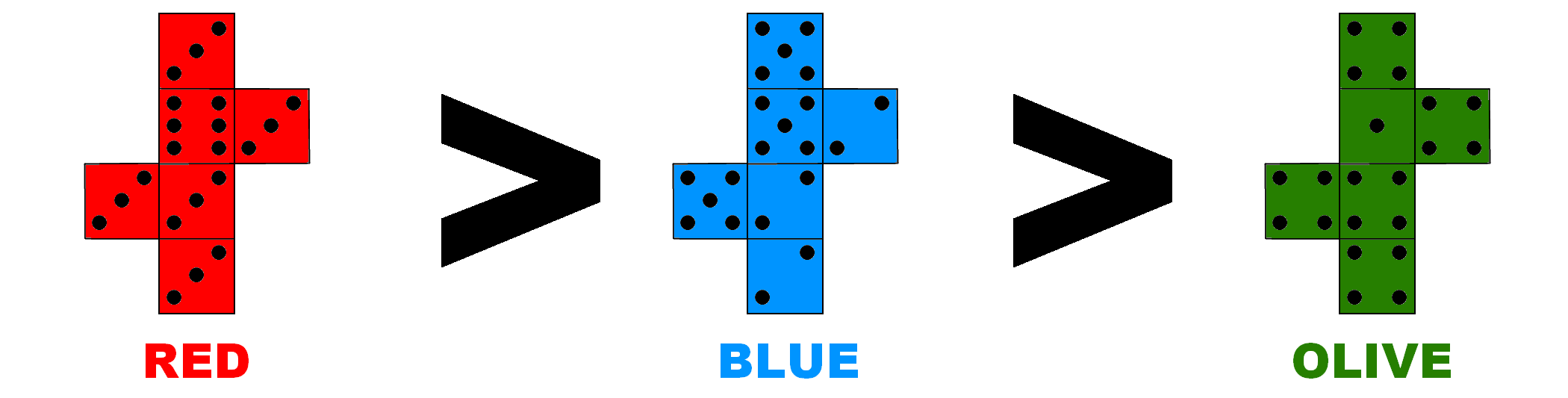If this is the case we call the dice `transitive' because the winning property transfers via the die in the middle, the Blue die.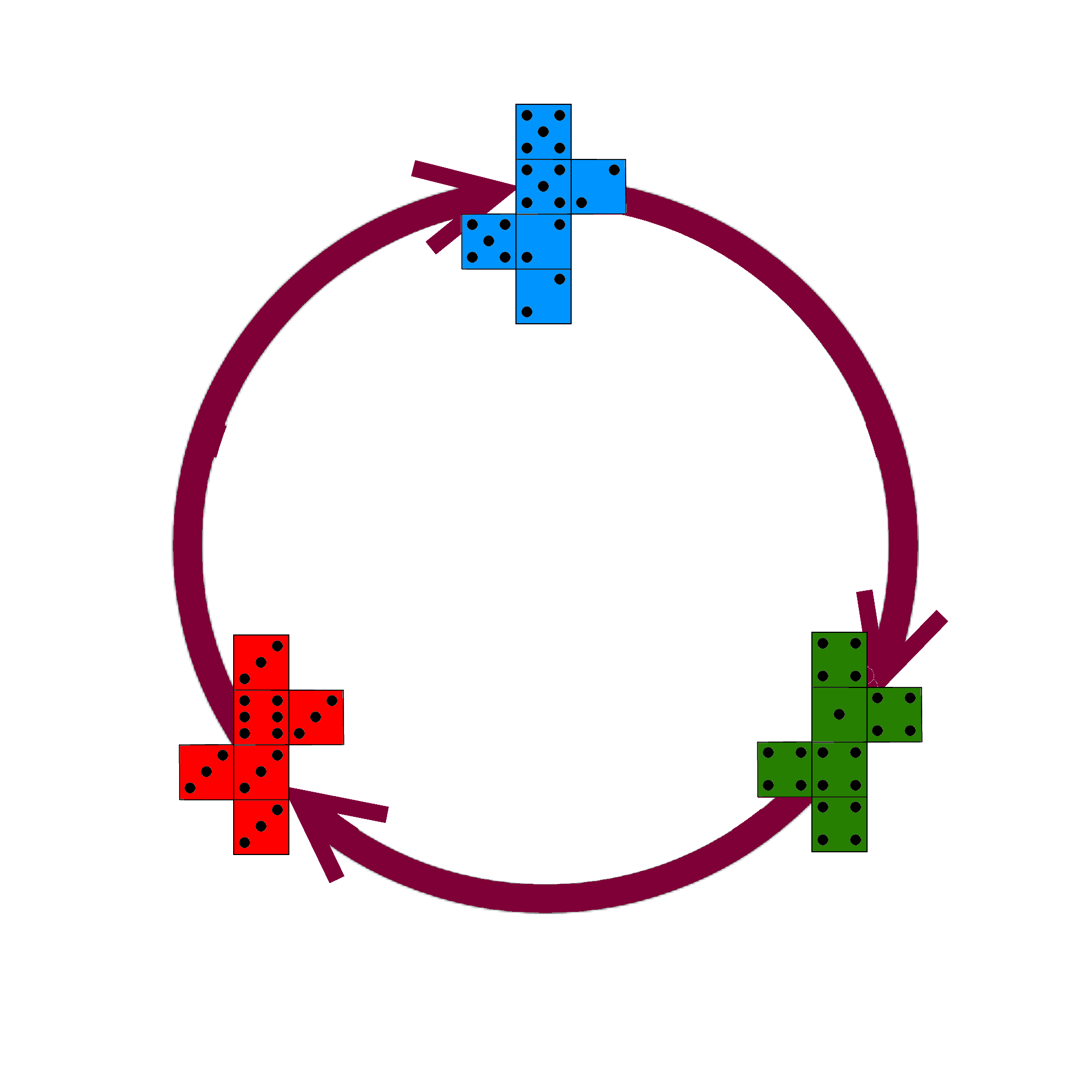However this is not the case. In fact, the winning property goes round in a circle - like a game of `Rock, Paper, Scissors' - with the Red die beating the Blue die, the Blue die beating the Olive die, and the Olive die beating the Red die. There is no strong or weak die, so the dice are `non-transitive'. How can this be?

 Let's do an example calculation and show that, in the long run, the Red die has a better chance of beating the Blue die. Notice, when you roll the Red die there are two possible outcomes; you either roll a 3 or a 6. The probability of rolling a 3 is 5/6, while the probability of rolling a 6 is 1/6. On the other hand, the Blue die can either roll a 2 or a 5, each with a probability of 1/2. So in total, if we roll the Red die and the Blue die together, we have four possible outcomes. I can draw a tree diagram of all the possible outcomes. You find the probability of each outcome by  multiplying the probabilities along the diagram. For example, the probability of rolling a 5 with the Red die and a 2 with the Blue die is 5/6 x 1/2 = 5/12. If I want to find the probability that the Red die  wins I add up the probabilities of all the outcomes where the Red die beats the Blue die. So in this case, the probability the Red die beats the Blue die is 7/12 - importantly, this is more than 1/2.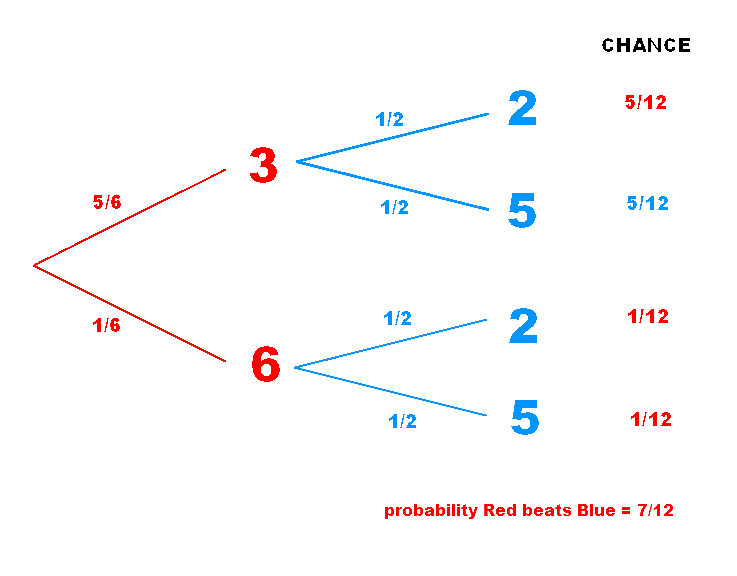In the same way it can be shown that the Blue die beats the Olive die, and then remarkably the Olive die beats the Red die. Here, you can remember the order since the colours are in increasing word-length, until it wraps back to Red.

So, as long as your opponent picks first, you will always be able to pick a die with a better chance of winning, with the average winning probability being around 62%    .

This set of three non-transitive dice are particular special because they are the optimum set of three dice. In other words, we have maximised the lowest winning probability. But that is not the only surprising thing about these particular dice.

## Double Whammy

After a few defeats your friend may have become suspicious, but all is not lost. Once you've explained how the dice beat each other in a circle, challenge your friend to one more game.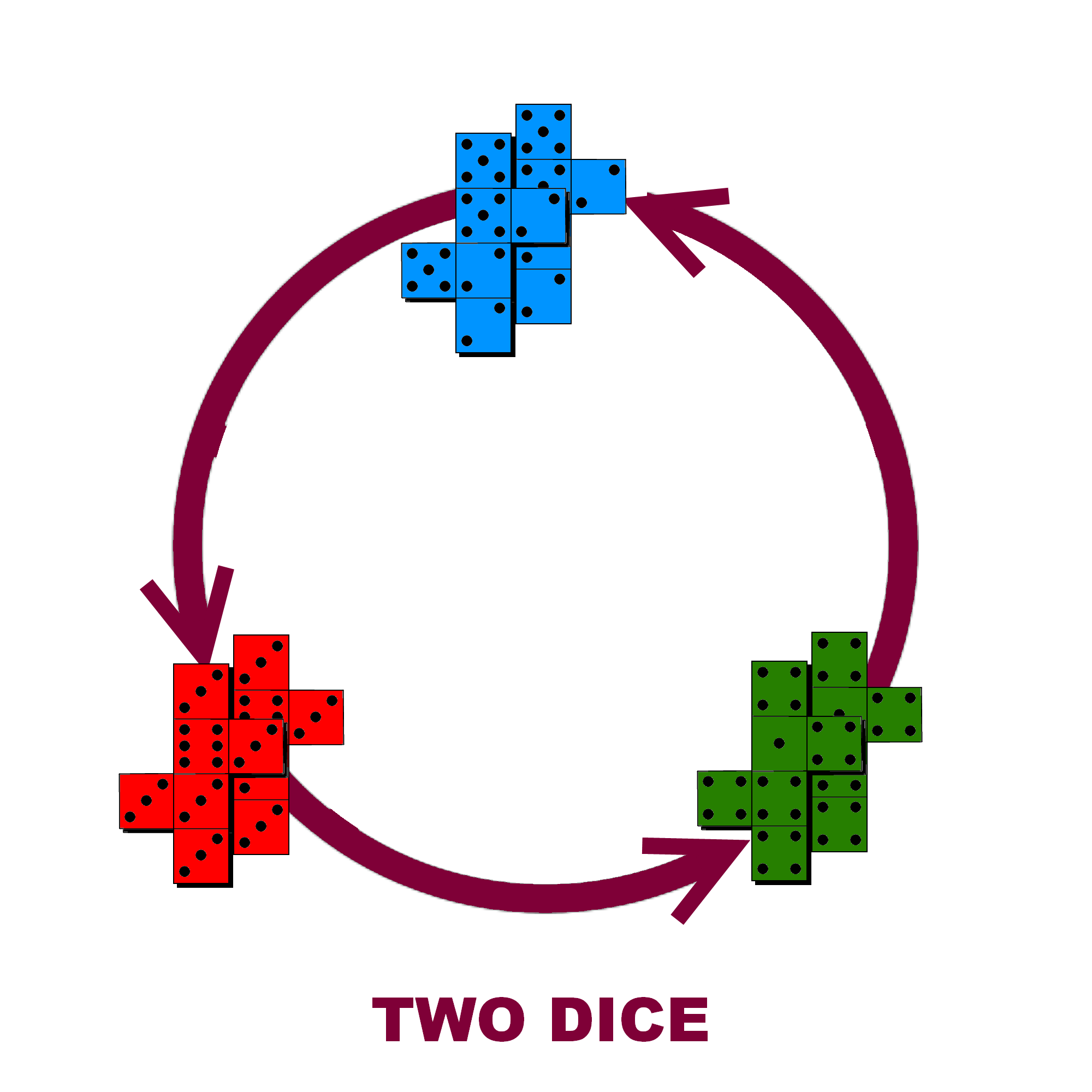This time you will choose first, in which case your opponent should be able to pick a die with a better chance of winning. But let's increase the stakes, and increase the number of dice. This time each player rolls two of his chosen die, so that the player with the highest total wins. Maybe two dice means your opponent has just doubled their chances of winning. But not so because, amazingly, with two dice the order of the chain flips! In other words, the chain reverses so the circle of victory now becomes a circle of defeat. Now,  the Red die beats the Olive die, the Olive die beats the Blue die, and the Blue die beats the Red die. Allowing you to win the game again!  The average winning probability with two dice is around 57%   . A word of warning though; although the probability that the Red die beats the Olive die is greater than 1/2, it is a slim victory. In the short term, say less than 20 rolls, the effect is closer to 50-50, so you will still need some luck on your side!

## Efron Dice

The idea for non-transitive dice has been around since the early 1970s  . However, the remarkable reversing property is not true for all sets of non-transitive dice, for you do need to pick your values carefully. For example, here is another famous set of non-transitive dice; it is a set of four non-transitive dice known as `Efron Dice' and invented by the American statistician Brad Efron: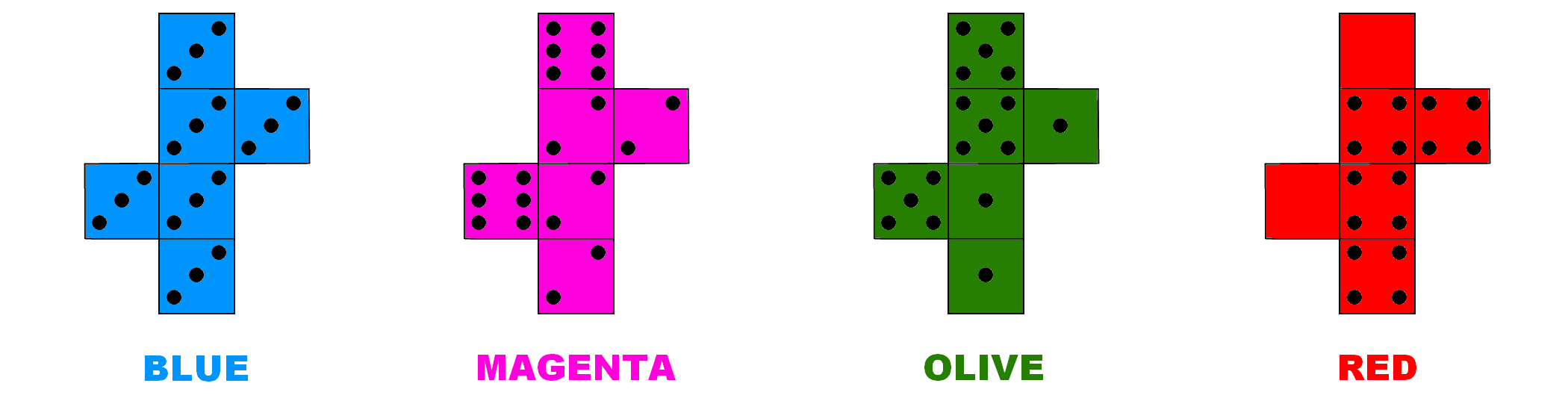This time the dice use values 0 to 6. Each die has values:

Blue: 3 3 3 3 3 3
Magenta: 2 2 2 2 6 6
Olive: 1 1 1 5 5 5
Red: 0 0 4 4 4 4

 As before, the dice form a circle where the Blue die beats the Magenta die, the Magenta die beats the Olive die, the Olive die beats the Red die, and the Red die beats the Blue die, and they each do so with a probability of 2/3. This time I have ordered the colours alphabetically. Efron Dice are optimal, in the sense that the lowest winning probability achieves the theoretical upper bound. We also have two pairs of dice opposing each other on the circle. In fact the Magenta die beats the Red die, but  the Blue die and the Olive die are equal, with each having a 50% chance of winning, and neither die dominating  . Unfortunately, the player picking the Blue die will not have a very exciting game, with all the values being the number 3. Also, this chain does not display the remarkable property of flipping when you double the number of dice .It is said the successful American investor Warren Buffett is a fan of non-transitive dice. When he challenged his friend Bill Gates to a game, with a set of Efron dice, Bill became suspicious and insisted Warren choose first. Maybe if Warren had chosen a set with a reversing property he could have beaten Gates - he would just need to announce if they were playing a one die or two dice version of the game after they had both chosen.

## Three Player Games

I wanted to know if it was possible to extend this to make a three player game, a set of dice where two of your friends may pick a die each, yet you can pick a die that has a better chance of beating both opponents - at the same time!It turns out there is a way. The Dutch puzzle inventor M. Oskar van Deventer  came up with a set of seven non-transitive dice, with values from 1 to 21  . Here two opponents may each choose a die from the set of seven, and yet there will be a third die with a better chance of beating each of them. The probabilities are remarkably symmetric with each arrow on the diagram illustrating a probability of 5/9. This means we can play two games simultaneously, but there is still the question of whether you can beat your two opponents at the same time.  If the dice were regular fair dice, with two competing dice having a 50-50 chance of victory (ignoring draws), then the chance of beating two opponents at the same time would stand at just over 25%. It isn't exactly 25% because beating one player is not independent of beating the other player - when you roll a high number you are likely to beat both! However, these dice are not fair. Even though you have the advantage against both opponents, beating both players is still a challenge, with the probability of doing so standing around 39%. This set of seven dice form a complete directed graph. In the same way, a four player game would require 19 dice. It is not known if such a set exists.

However, if it is possible to construct a set of non-transitive dice with a reversing property, then it might be possible to have a three player game with fewer than seven dice. And potentially such a set might improve our chances of beating two players at the same time. I have devised such a set below.

## Grime Dice

Here is a set of five non-transitive dice:These dice use values from 0 to 9, as follows;

Red: 4 4 4 4 4 9
Yellow: 3 3 3 3 8 8
Blue: 2 2 2 7 7 7
Magenta: 1 1 6 6 6 6
Olive: 0 5 5 5 5 5

 This set of five dice is similar to other sets of dice we have seen, in that we have a chain where Blue>Magenta>Olive>Red>Yellow>Blue. However, we also have a second chain, where Red>Blue>Olive>Yellow>Magenta>Red. Notice the first chain is ordered alphabetically, while the second chain is ordered by word-length. In general, the chain in alphabetical order is stronger than the chain in order of word-length. But if your friend discovers you using one chain, you can switch to the other. Overall, the average winning probability for one die is 63%   . If our original set of three non-transitive dice was like a game of `Rock, Paper, Scissors', this diagram is closer to the related, but more extreme, non-transitive game `Rock, Paper, Scissors, Lizard, Spock'  .But not only that, because if you consider subsets of these five dice you will find other non-transitive chains! In particular, the first three dice in the chain ordered by word- length; that's Red, Blue and Olive; is a copy of the optimal set of three non-transitive dice that I describe above. So with this set of five dice you get the optimal set of three dice for free! In fact, any three consecutive dice in the chain ordered by word-length makes a set of three non- transitive dice.

And not only that, because any four consecutive dice in the alphabetical chain makes a set of four non-transitive dice. The first four in this chain; that's Blue, Magenta, Olive and Red; is not a copy of the optimal set of four dice, but they do have the same average winning probability as Efron dice.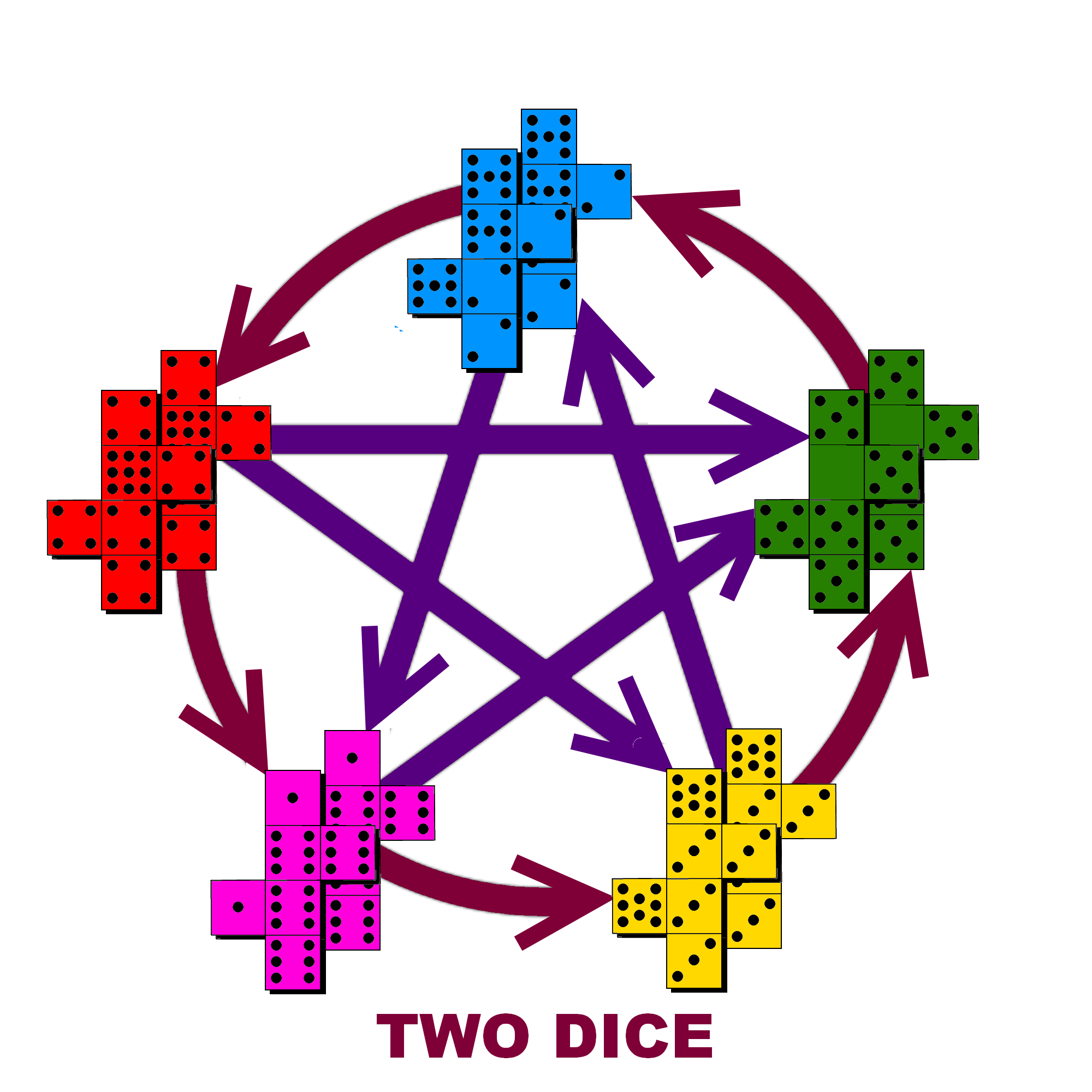With two dice the chain ordered by word-length now flips so Magenta>Yellow>Olive>Blue>Red>Magenta. On the other hand, the alphabetical chain stays the same - with one exception, the Red-Olive arrow reverses. This is to be expected since Red, Blue and Olive form a copy of the optimal set of three. With two dice, the chain ordered by word-length is stronger than the alphabetical chain. The average winning probability for two dice is 59%  . However, the probability that two Red dice beats two Olive dice is close to 50%. If we treat these dice as equal we can now play two games simultaneously. Invite two opponents to pick a die each, but do not volunteer whether you are playing a one die or two dice version of the game. If your opponents pick two dice that are next to each other alphabetically then play the one die version of the game. Use the diagram for the one die game to pick a die that can beat each opponent. If your opponents pick two dice that are next to each other in the chain ordered by word-length, then play the two dice version of the game. Again, use the diagram for the two dice game (that treats Red and Olive as equal) to pick a die that can beat each opponent.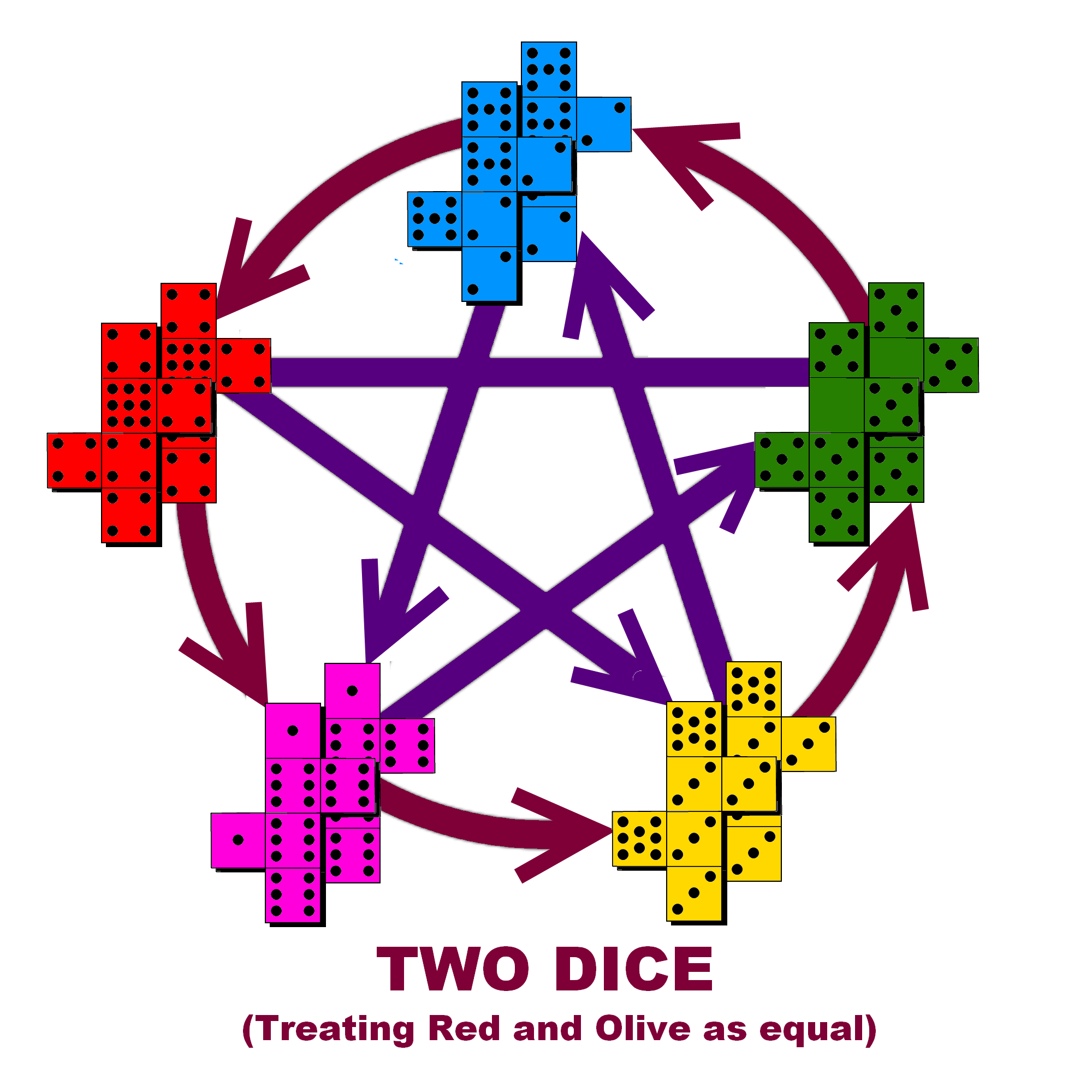## A Gambling Game

 But, can we expect to beat the two other players at the same time? Well, we have certainly improved the odds, with the average probability of beating both opponents now standing around 44% - a 5% improvement over Oskar Dice, and a 19% improvement over fair dice!   So, if the odds of beating two players isn't over 50% then how do we win? Consider the following gambling game: Challenge two friends to a dice game, where you will play your two opponents at the same time. If you lose you will give your opponent £1. If you win your opponent gives you £1. So, if you beat both players at the same time you win £2; if you lose to both players you lose £2; and if you beat one player but not the other then your net loss is zero. You and your friends decide to play a game of 100 rolls. If the dice were fair then each player will expect to win zero - each player wins half the time and loses half the time. However, with Oskar Dice, you should expect to beat both players 39% of the time, and lose to both players 28% of the time, which will give you a net profit of £22. But even better, with Grime Dice, you should expect to beat both players 43.8% of the time, but only lose to both players 22.7% of the time, giving you an average net profit closer to £42! (And possibly the loss of two former friends). This set is the best set of five non-transitive dice with these properties that I have found.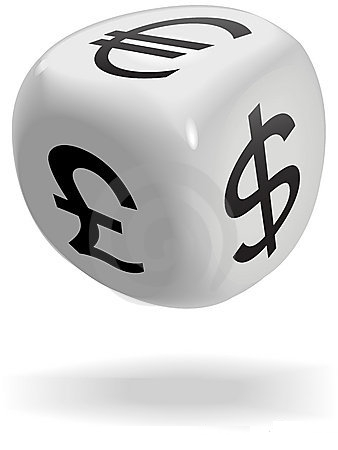I invite you to try out these games yourself . They are easy to make by either writing on blank dice, or modifying some old dice. They are also inexpensive to buy and you can get the set of five Grime Dice from Maths Gear. Remember, the set of five Grime dice contains subsets of three and four non-transitive dice for free, including the optimal set of three. Or, you can buy the optimal set of three separately.

Try them out on your friends and enjoy your successes and failures!

Footnotes:

 Other examples of transitivity: If a, b and c are real numbers then if a > b and b > c, then we know a > c. For example, if 9 > 4.6 and 4.6 > 5/3 then 9 > 5/3.
If a, b and c are integers such that a stictly divides b (with no remainder) and b strictly divides c, then we know a strictly divides c. For example, if 3|15 and 15|60 then 3|60.
The last two relations are transitive. On the other hand, if a,b and c are integers such that a+b > 7 and b+c > 7, we cannot deduce that a+c > 7. This relation is not transitive.

 In full the probabilities are: P(Red > Blue) = 7/12, P(Blue > Olive) = 7/12 and P(Olive > Red) = 25/36.

 Tim Rowett introduced this set of non-transitive dice at the Gathering for Gardner V (2002).

 This time the full probabilities are: P(Red > Olive) = 671/1296 (a slim victory!), P(Olive > Blue) = 85/144, and P(Blue > Red) = 85/144.

 Gardner, M. "Mathematical Games: The Paradox of the Nontransitive Dice and the Elusive Principle of Indifference." Sci. Amer. 223 , 110-114, Dec. 1970.

 Efron Dice: P(Blue > Magenta) = 2/3, P(Magenta > Olive) = 2/3, P(Olive > Red) = 2/3, P(Red > Blue) = 2/3, P(Blue > Olive) = 1/2 and P(Magenta > Red) = 5/9

 The probabilities for two sets of Efron Dice are: P(Blue > Red) = 5/9, P(Magenta > Blue) = 5/9, P(Magenta > Olive) = 5/9, P(Olive > Red) = 5/9, P(Magenta > Red) = 11/27, P(Red > Magenta) = 0, P(Magenta = Red) = 16/27 (a draw), P(Blue > Olive) = 1/4, P(Olive > Blue) = 1/4, P(Blue = Olive) = 1/2. There is no chain like before.

 Mathematical Association of America on Oskar dice: http://www.maa.org/editorial/mathgames/mathgames_07_11_05.html

 Grime Dice, one die version: P(Red > Blue) = 7/12, P(Blue > Olive) = 7/12, P(Olive > Yellow) = 5/9, P(Yellow > Magenta) = 5/9, P(Magenta > Red) = 5/9. P(Blue > Magenta) = 2/3, P(Magenta > Olive) = 13/18, P(Olive > Red) = 25/36; P(Red > Yellow) = 13/18, P(Yellow > Blue) = 2/3.

 Home of Rock, Paper, Scissors, Lizard, Spock: http://www.samkass.com/theories/RPSSL.html Or if that isn't extreme enough, how about Rock, Paper, Scissors with 101 gestures; RPS-101 http://www.umop.com/rps101.htm

 Grime Dice, two dice version: P(Magenta > Yellow) = 16/27, P(Yellow > Olive) = 56/81, P (Olive > Blue) = 85/144, P(Blue > Red) = 85/144, P(Red > Magenta) = 56/81. P(Blue > Magenta) = 5/9, P(Magenta > Olive) = 7/12, P(Olive > Red) = 625/1296(*), P (Red > Yellow) = 7/12, P(Yellow > Blue) = 5/9.
(*)This is a slim defeat! However, when rounded to the nearest whole number, the expected number of wins is the same as 50% for anything less than 28 trials. Also, 95% of the time we expect the number of wins to be plus/minus two standard deviations from the mean. When we take this into account, the effect is little different from 50%.

 In the ideal three player game, we would like the average probability of beating two players to be over 50%. This would mean, on average, each winning die should beat another with a probability of over 70%. It has been shown that for three non-transitive dice, the die with the smallest winning probability has an upper bound, namely the Golden Ratio Conjugate Φ = 0.618. The upper bound may not necessarily be obtainable, and it will depend on the number of sides the dice have. You will come closest to the upper bound when the number of sides is a Fibonacci number. Rowett Dice are the optimum set of three six-sided dice. For four non-transitive dice the upper bound is 2/3 - making Efron dice optimal in this sense, since the die with the smallest winning probability obtains its upper bound. For five dice the upper bound is 0.692. This sequence of upper bounds increases with the number of dice and converges to 3/4. Naturally, if the weakest die achieves the upper bound then the average winning probability will be even higher. References; The Paradox of Nontransitive Dice, Richard P. Savage, Jr. The American Mathematical Monthly, Vol. 101, No. 5 (May, 1994), pp. 429-436 http://www.jstor.org/stable/2974903 and; S. Trybula, On the paradox of n random variables, Zastos. Mat. 8 (1965), 143-154.

 For the very keen, here's a set to try that uses mathematical constants: A: 1 1 1 1 1 π ; B: Φ Φ Φ e e e; C: 0 φ φ φ φ φ ; where  e is Euler's Number, φ is the Golden Ratio and Φ is the Golden Ratio Conjugate. Do these dice form a non-transitive set? What about with two dice, does the order reverse as before?

This Web Page Created with PageBreeze Free HTML Editor